# Dielectric Properties of Insulating Materials (Formula & Constant)

We can first go through the description of dielectric materials. It actually does not conduct electricity. They are insulators having very low electrical conductivity. So we have to know the difference between dielectric material and insulating material. The difference is that insulators block the flow of current but the dielectrics accumulate electrical energy. In capacitors, it performs as electrical insulators.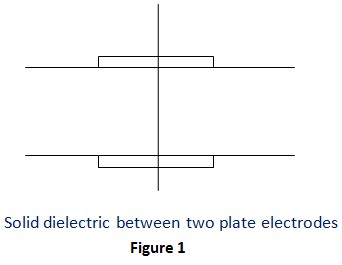Next, we can come to the topic. The dielectric properties of insulation include breakdown voltage or dielectric strength, dielectric parameters like permittivity, conductivity, loss angle and power factor. The other properties include electrical, thermal, mechanical and chemical parameters. We can discuss the main properties in detail below.

## Dielectric Strength or Breakdown Voltage

The dielectric material has only some electrons in normal operating condition. When the electric strength is increased beyond a particular value, it results in breakdown. That is, the insulating properties are damaged and it finally becomes a conductor. The electrical field strength at the time of breakdown is called breakdown voltage or dielectric strength. It can be expressed in minimum electrical stress that will result in breakdown of the material under some condition.

It can be reduced by ageing, high temperature and moisture. It is given as
Dielectric strength or Breakdown voltage =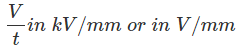V→ Breakdown Potential.
t→ Thickness of the dielectric material.
Relative permittivity
It is also called as specific inductive capacity or dielectric constant. This gives us the information about the capacitance of the capacitor when the dielectric is used. It is denoted as εr. The capacitance of the capacitor is related with separation of plates or we can say the thickness of dielectrics, cross sectional area of the plates and the character of dielectric material used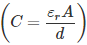. A dielectric material having high dielectric constant is favoured for capacitor.
Relative permeability or dielectric constant =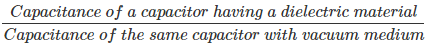We can see that if we substitute air with any dielectric medium, the capacitance (capacitor) will get improved. The dielectric constant and dielectric strength of some dielectric materials are given below.

 Dielectric material Dielectric Strength(kV/mm) Dielectric Constant Air 3 1 Oil 5-20 2-5 Mica 60-230 5-9

Table no.1

## Dissipation Factor, Loss Angle and Power Factor

When a dielectric material is given an AC supply, no power utilization takes place. It is perfectly achieved only by vacuum and purified gases. Here, we can see that the charging current will head the voltage applied by 90o which is shown in figure 2A. This implies there is no loss in power in insulators. But most of the cases, there is a dissipation of energy in the insulators when alternating current is applied. This loss is known as dielectric loss. In practical insulators, the leakage current will never lead the voltage applied by 90o (figure 2B). The angle formed by the leakage current is the phase angle (φ). It will be always less than 90. We will also get the loss angle (δ) from this as 90- φ.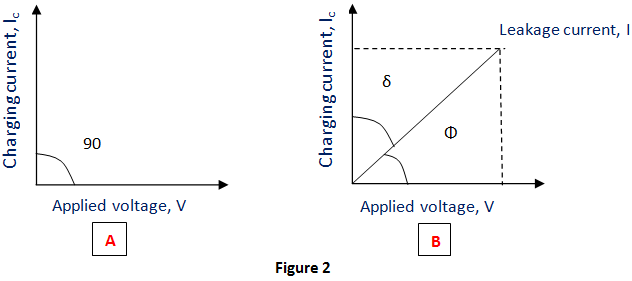The equivalent circuit with capacitance and resistor in collateral (parallel) are represented below.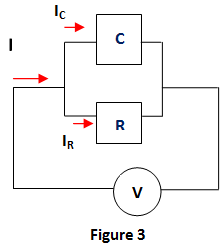From this, we will get the dielectric power loss as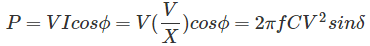X → Capacitive reactance (1/2πfC)
cosφ → sinδ
In most cases, δ is small. So we can take sinδ = tanδ.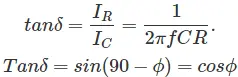So, tanδ is known as power factor of dielectrics.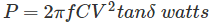The importance of the knowledge of the properties of dielectric material is in the scheming, manufacturing, functioning and recycling of the dielectric (insulating) materials and it can be determined by calculation and measurement.

Want To Learn Faster? 🎓
Get electrical articles delivered to your inbox every week.
No credit card required—it’s 100% free.